International Journal of Oil, Gas and Coal Engineering
Volume 4, Issue 1-1, February 2016, Pages: 1-7

Pressure Transient Analysis for Finite Conductivity Multi-staged Fractured Horizontal Well in Fractured-Vuggy Carbonate Reservoirs

Mingqiang Wei1, Yonggang Duan1, Xuemei Zhou2, Quantang Fang1

1State Key Laboratory of Oil and Gas Reservoirs Geology and Exploitation, Southwest Petroleum University, Chengdu, Sichuan Province, PR China

2Shell China Exploration and Production Co, Chengdu, Sichuan Province, PR China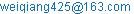(Mingqiang Wei)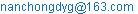(Yonggang Duan)

*Corresponding author

Mingqiang Wei, Yonggang Duan, Xuemei Zhou, Quantang Fang. Pressure Transient Analysis for Finite Conductivity Multi-staged Fractured Horizontal Well in Fractured-Vuggy Carbonate Reservoirs. International Journal of Oil, Gas and Coal Engineering. Special Issue: Academic Research for Multidisciplinary. Vol. 4, No. 1-1, 2016, pp. 1-7. doi: 10.11648/j.ogce.s.2016040101.11

Received: August 12, 2015; Accepted: May 17, 2016; Published: June 30, 2016

Abstract: Transient pressure analysis has been considered as a robust method to identify the flow behaviors, estimate reservoir parameters and hydraulic fracturing parameters. Compared with conventional clastic reservoirs, complex pore systems in fractured–vuggy carbonate reservoirs are posing significant research challenges. Although pressure transient analysis(PTA) models for different style wells in carbonate reservoirs have been widely studied, limited study has been conducted for multi-fractured horizontal well (MFHW) in these reservoirs. Thus, this paper is an investigation on PTA for MFHW of fractured-vuggy carbonate reservoirs. Based on source function theory, mirrors reflection and superposition principle,, the finite conductive MFHW’s bottom-hole pressure solution for fractured-vuggy carbonate reservoirs is obtained by the Laplace transform and the Stehfest inversion method. Log-log type curves are drawn by numerical algorithms and eight flow regimes are identified. Finally the influences of sensitivity parameters such as fracture number, fracture spacing, half length of fracture, storativity ratio, inter-porosity flow coefficient on unsteady flow behaviors of MFHW are discussed in depth.

Keywords: Fractured-Vuggy Carbonate Reservoir, Multi-fractured Horizontal Well, Finite Conductive, Transient Pressure

Contents

1. Introduction

Compared with conventional clastic reservoirs, fractured-vuggy carbonate reservoirs are composed of matrix, fractures, vugs systems. Among these systems, the properties of porosity, permeability and fluid transport behaviors are quite different, which brings a big challenge to study the fluids flow and transport behaviors in these reservoirs , , .

In recent decades, many scholars have made some efforts to study the fluids flow and transport behaviors in fractured–vuggy reservoirs. G. Fuentes-Cruz et al.  studied the transient pressure and production decline curve behaviors for partially penetrating wells completed in fractured-vuggy reservoirs. Camacho-Velázquez et al.  proposed an unsteady flow analysis model for carbonate reservoirs, and analyzed the transient pressure and production decline curves. Subsequently, the transient pressure of fractured well in fractured-vuggy reservoirs is investigated by Cai and Jia . Cheng et al.  used triple media model to establish the well testing model for multi-branch horizontal wells. And the numerical well testing method for carbonate reservoirs is studied by Corbett et al. . However this method is limited for isolated cave. Gulbransen et al.  propsed a multi-scale mixed finite element method for modeling of vuggy and naturally fractured reservoirs. Wu et al. , ,  proposed a triple-continuum model to describe flow in fractured rock & naturally fractured vuggy reservoirs. Ren et al.  proposed a well testing model of inclined well for fractured-vuggy carbonate reservoirs. Jia et al.  developed a flowing model of well test analysis for porous-vuggy carbonate reservoirs. After that, a analytical model for wormholes in fractured–vuggy carbonate reservoirs is developed by Wang et al. . Chen et al.  used boundary element method for modeling of fractured-vuggy reservoirs with large-scale caves and obtained the PTA type curves. Guo et al.  and Nie et al.  investigated the flow behavior for modeling horizontal well production in fractured-vuggy carbonate reservoirs. However, these aforementioned models were mainly developed for vertical wells, fractured wells and horizontal wells.

According to above mentioned models in fractured-vuggy carbonate reservoirs, two main models are used to describe and study the flow process in fractured-vuggy carbonate reservoirs: (1) triple porosity and single permeability model , . The model considers the vug system as a continuum medium, treats fracture system as pathways directly connected to the wellbore, and considers the matrix and vug systems connect to the fracture system. And the inter-porosity flows are considered from the matrix and vug systems to the fracture system; (2) triple porosity and dual permeability model . This model also considers the vug system as a continuum medium, treats fracture system and vug system as pathways directly connected to the wellbore and considers the inter-porosity flows to occur between matrix, fracture and vug systems.

Although series of progress onPTA in fractured-vuggy carbonate reservoirshave been made by using the two models, little study on MFHW of PTA has been conducted in fractured-vuggy carbonate reservoirs. Fortunately, many studies on pressure behaviors of MFHW in clastic and unconventional reservoirs have been performed in recent 20 years , , , , , , , which can guide to study PTA of MFHW in fractured-vuggy carbonate reservoirs.

To extend the PTA theory into MFHW in fractured-vuggy carbonate reservoirs, a triple porosity and single permeability model is used in this paper to investigate the transient pressure behavior of finite conductive MFHW. Based on Source function theory, mirrors reflection and superposition principle, the finite conductive MFHWs bottom-hole pressure solution is obtained by Laplace transform and Stehfest inversion methods. Type curves are drawn by numerical simulations and eight flow regimes are identified. Finally the sensitivity parameters were discussed in depth.

2. Physical Model

Generally speaking, fractured-vuggy carbonate reservoir consists of matrix, fractures and vuggy (Fig. 1). Fluids in the reservoir would flow into wellbore under the pressure drop between wellbore and reservoir pressures, and the triple porosity and sequential single permeability of MFHW flow scheme is shown in Fig. 3.a) Real core photo of ractured-vuggy reservoir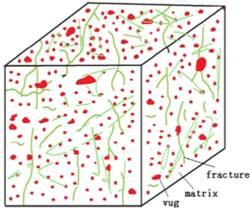(b) Physical scheme of Naturally fractured-vuggy reservoir scheme

Fig. 1. Naturally fractured-vuggy reservoir scheme.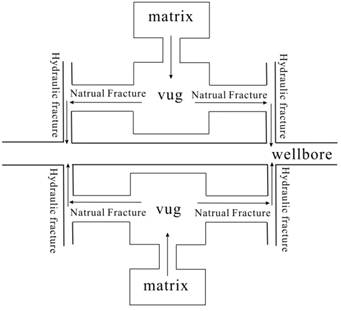Fig. 2. Triple porosity and sequential single permeability of MFHW flow scheme in fractured-vuggy carbonate reservoirs

Assumptions are made: the reservoir is homogenous and isotropic with the same thickness h, and the initial reservoir pressure is equal to pi; reservoir consists of fractures, vugs and matrix; the natural fractures are the main flow channel; fluids in matrix act as a source flowing into vuggy, while fluids in vug flow into NF; the interporosity flow process is considered as pseudo -steady flow; the horizontal well is in the center of the reservoir; and the completion of the horizontal well is casing perforation, meanwhile the perforation spots are only placed at fractured points; hydraulic fractures are perpendicular to the horizontal wellbore and penetrate the whole reservoirs; the fracture number is m and the hydraulic fracture is symmetrical with borehole; the fracture half length is xf; while the fracture width is ignored; the viscosity and compressibility of the rock and the fluids are considered as constant, and isothermal and Darcy flow are also considered; the gravity is ignored.

3. Mathematical Model and Solution

3.1. Mathematical Model

According to the physical model assumptions, the flow equation in porous fractured-vuggy carbonate reservoirs is established as follows:

The reservoir governing equation is: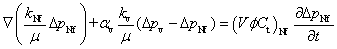(1)

The formula of interporosity flow from vug sto NFs is: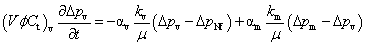(2)

The inter flow equation from matrix to vugs is: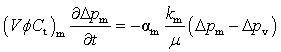(3)

Taking the Laplace transform of Eqs.(1)~(3), Eqs.(4)~(6) can be obtained, respectively:(4)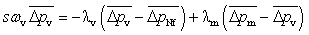(5)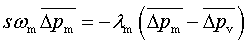(6)

Combining Eqs.(5) ~(6) and the following dimensionless definitions, Eq. (4) can be written as:(7)

In Eq.(7), the expression of u is:(8)

All the Dimensionless definitions in the paper are as below:

Dimensionless distance: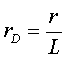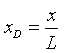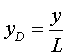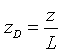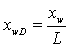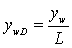Dimensionless wellbore storage coefficient: Dimensionless time: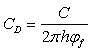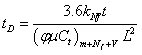Dimensionless pressure: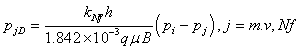The parameters in Eq. (8) are defined as follows:

Vug storage capacity ratio: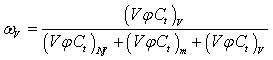Interporosity flow coefficient from vugs to NFs: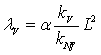Matrix storage capacity ratio: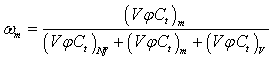Interporosity flow coefficient from matrix to vugs: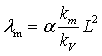Based on Eqs.(7) and (8), the flow equation is established by utilizing the point source function and boundary conditions:(9)

Furthermore, based on Eq. (9), the continuous point source solution,  of closed top and bottom borders of reservoirs can be obtained by the mirrors reflection and the superposition principle:(10)

3.2. Solution of the Model

The pressure transient solution for MFHW in naturally fractured-vuggy reservoirs is obtained by discretizing fractures (Fig.3) and the use of source function method.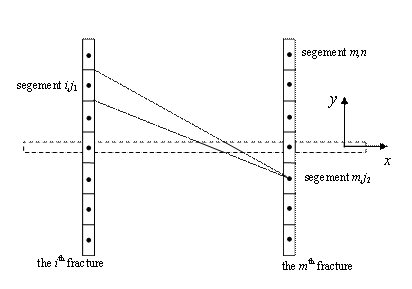Fig. 3. Schematic of discretion of fractures.

The dimensionless pressure solution of the discrete segment(i,j) of the infinite conductive fracture MFHW with infinite boundary can be obained by integrating Eq.(10) along z over the interval of 0 to h and then along xw over the interval of xi,j to xi,j+1.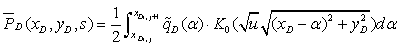(11)

In Eq.(11)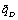is the Laplace transform of qD(xD, tD). By writing Eq.(11) for midpoints for all discrete segments, we can get m×n equations with (m×n+1) unknowns which are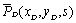and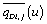. To obtain the pressure responses, one more equation can be written by assuming the flow rate is constant: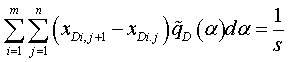(12)

Considering the skin effect of the fracture wall, the relationship between fracture pressure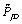and formation pressure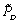can be written as: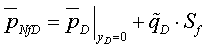(13)

Since many proppants will still exist after the well fracturing, which leads to flow resistance in the hydraulic fractures, several previous transient pressure analysis of MFHW assuming infinite conductivity of fractures is not realistic. Thus, the finite conductive fractures of MFHW in carbonate reservoirs is considered

The flow equations  of finite conductive hydraulic fracture ignoring the liquid compressibility can be written: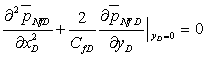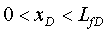(14)

The boundary conditions are given as follows: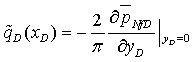(15)(16)

In Eq.(16), the dimensionless conductive coefficient is CfD=(kfwf)/(kLf), kf is the fracture permeability, wf is the fracture width.

Combined with boundary conditions of Eqs.(15)~(16), Eq.(14) can be obtained after integrating.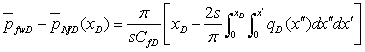(17)

Eq. (17) can be rewritten based on Eqs. (11) and (13):(18)

In order to get approximate expansion of Eq.(17), a fracture wing is divided into n segments by discrete fracture mechanism (see Fig3). Assuming the flow rate of each segment is uniform distributed, Eq.(18) can be expanded by discrete approximate method.(19)

Where x=LfD/n, xD,j is the center of the jth segment, xD,i is the starting point of ith segment, xD,i+1 is the end point of ith segment. n equations for n discrete segments can be established. According to the assumption of constant flow rate, the constraint of flow rate can be written :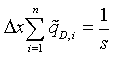(20)

Thus the n+1 equations are composed,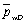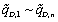can be calculate by numerical method. When the production rate is constant, wellbore storage and skin can be easily added to Eq.(19) as a final step according to Duhamels principle :(21)

4. Analyses of Transient Pressure Behaviors

The unsteadypressure characteristic type curves (Fig.4) of finite conductive MFHW for triple porosity and sequential single permeability in fractured-vuggy reservoirs are drawn by convertingback to pwD based on Stehfest numerical inversion .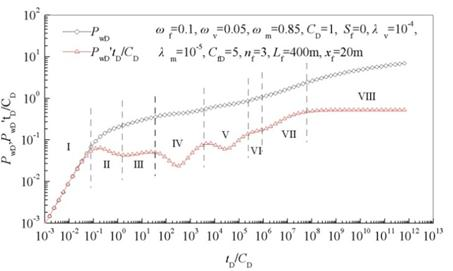Fig. 4. Transient pressure characteristic curves of finite conductive MFHW for triple porosity and sequential single permeability in fractured-vuggy reservoirs.

Fig4 shows that the transient pressure behavior can be divided into eight flow regimes. I: Wellbore storage regime. Curves for both pressure and pressure derivative are in a unite slope trend. II: Transient flow regime between well storage and early linear flow which presents a hump. III: Early linear flow regime. The pressure derivative curve is a line with a slope of 0.25; IV: Inter-porosity flow regime from vug to NF. Because vug permeability is higher than NF permeability; there is a characteristic dip. V: Inter-porosity flow regime from matrix to vug. The pressure derivative curve is also a dip controlled by interporosity flow from matrix system to vug system. VI intermediate-time pseudo-radial flow regime around individual fractures which is influenced by the distance between hydraulic fractures. VII: Compound linear flow which represents the linear flow normal to horizontal well axis. VIII: Compound radial flow regime which indicates the pressure of matrix, fracture, and vug systems have arrived at a dynamic balance. The derivative responses of pressure shows a flat behavior .

As is known from Eq.(19), the MFHW well testing typical curves in carbonate reservoirs are mainly influenced by fracture number, distance between fractures, half length of fracture, storage and inter porosity flow.

Fig.5 illustrates the effects of fracture number on the transient pressure of finite conductive MFHW for triple porosity and sequential single permeability in fractured-vuggy reservoirs. The typical curve characteristics show that with the increase of fracture number, the location of the pressure and pressure derivative curve becomes lower among II~VII regime. The main reason is that the interference between fractures will be stronger as the fracture number increases with other parameters(such as wf, wv, wm, Lf ,xf ,λv,λm et al.) being constant.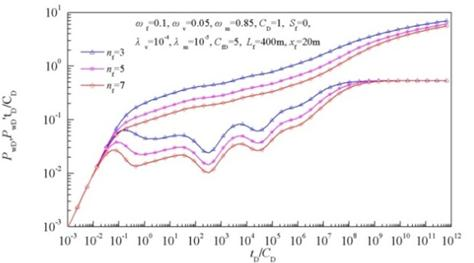Fig. 5. Effect of fracture number on the transient pressure of finite conductive MFHW for triple porosity and sequential single permeability in fractured-vuggy reservoirs.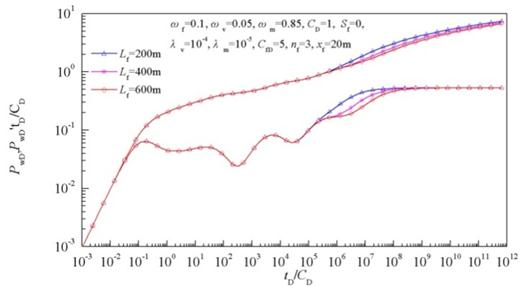Fig. 6. Effect of fracture distance on the transient pressure of finite conductive MFHW for triple porosity and sequential single permeability in fractured-vuggy reservoirs.

Fig. 6 presents how the fracture distance influences the MFHW well testing curves of triple porosity and sequential single permeability in fractured-vuggy reservoirs. From Fig6 we can see that when the distance between fractures increases, compound linear flow emerges later. The reason is that interference between fractures will weaken as the distance between fractures increases.

Fig.7 illustrates that the half length of fracture mainly affects the early linear flow regime and two inter-porosity flows. Meanwhile, it indicates that the longer the fracture length is, the lower the location of early linear flow regime and two inter-porosity flows regimes in the pressure and pressure derivative curve is.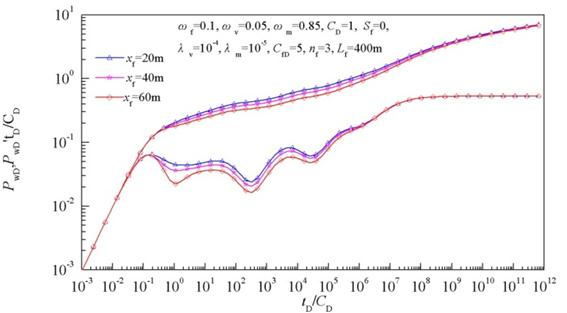Fig. 7. Effect of half length of fracture on the transient pressure of finite conductive MFHW for triple porosity and sequential single permeability in fractured-vuggy reservoirs.

Fig.8 and Fig.9 denote how the vug and matrix interporosity flow coefficient affect the MFHW well testing curves of triple porosity and sequential single permeability in fractured-vuggy reservoirs respectively. It shows that as the interporosity flow coefficient goes higher, the dig appears earlier(Fig.8). The vug interporosity flow coefficient influences the first dip, while matrix interporosity flow coefficient influences the second one(Fig.9). Meanwhile, if the value of parameters is inappropriate, the eight flow regimes of the transient pressure behavior of finite conductive MFHW for triple porosity and sequential single permeability in fractured-vuggy reservoirs will not fully emerge (Fig.8~Fig.10).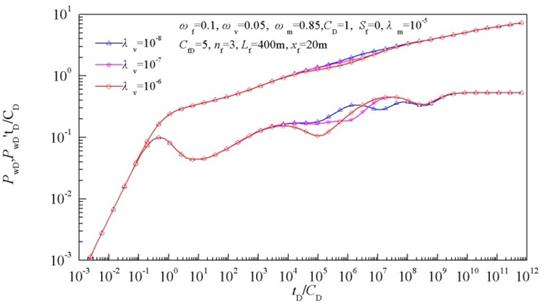Fig. 8. Effect of vug interporosity flow coefficient on the transient pressure of finite conductive MFHW for triple porosity and sequential single permeability in fractured-vuggy reservoirs.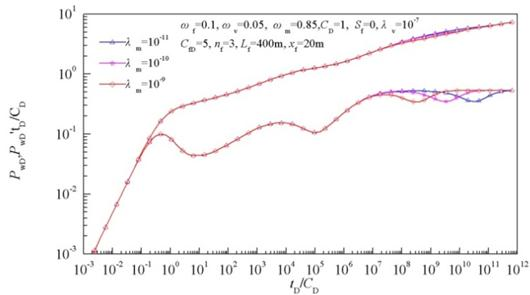Fig. 9. Effect of matrix interporosity coefficient on the transient pressure of finite conductive MFHW for triple porosity and sequential single permeability in fractured-vuggy reservoirs.

Fig.10 indicates the influences of storativity ratio on the dips depth. Since the total of reservoir is 1, if the storativity ratio of one system changes, another two systems will also change in response. Thus, the well testing curves are drawn with different vug and matrix storativity ratio while fracture storativity ratio keeps 0.1. Fig.10 shows that the first dip become deeper while the second dip become lower as the vug storativity and matrix storativity increases and decreases respectively.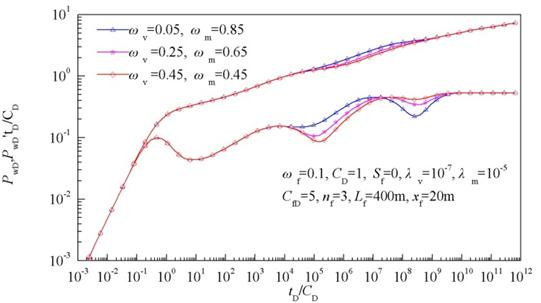Fig. 10. Effect of storativity ratio on the transient pressure of finite conductive MFHW for triple porosity and sequential single permeability in fractured-vuggy reservoirs.

5 Conclusions

1) Based on the Source function theory, the mirrors reflection and the superposition principle, the finite conductive MFHWs bottom-hole pressure solution for natural fractured-vuggy carbonate reservoirs is obtained.

2) Well testing typical curves of MFHW in natural fractured-vuggy carbonate reservoirs are drawn, adn the ones are characterized by eight flow regimes including wellbore storage regime, transient flow regime, early linear flow regime, inter-porosity flow regime of vug to NF, inter-porosity flow regime of matrix to vug, intermediate-time pseudo-radial flow regimecompound linear flow regime, and compound radial flow regime

3) The sensitivity parameters of MFHWs transient pressure well testing curves are discussed. The results indicate that fracture number affects the location of the pressure and pressure derivative curve among II~VII regimethe distance between fractures mainly influence the time compound linear flow emerges; fracture length has a primary effect on the location of the early linear flow regime and two inter-porosity flows regimesthe storativity ratio influences dips depth and the interporostiy flow mainly affects the time dip emerges.

Nomenclature

k=permeability(m2)

p= Pressure drop(MPa)

μ=oil viscosity(mPa.s)

Ct= oil compressibility(MPa-1)

Lf=distance between fractures(m)

nf=fracture number

xf=half length of fracture(m)

α=shape factor(m-2)

Φ=porosity(decimal)

w=storativity ratio

λ=interporosity flow coefficient

h= reservoir thickness(m)

t=time(hour)

V= volume(m3)

s=time in Laplace space

L=reference length(m)

xD= dimensionless x of discrete fracture segement

xwD= dimensionless center point’s x of discrete fracture segement

ywD= dimensionless center point’s y of discrete fracture segement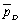=dimensionless pressure in Laplace space

Sf= skin of fracture wall

Subscripts

Nf=natrual fracture system

m=matrix system

v=vug system

i= the ith discrete segment of fracture

References

1. Abdassah D, Ershaghi I. Triple-porosity systems for representing naturally fractured reservoirs [J]. SPE Formation Evaluation, 1986, 1(02): 113-127.
2. Liu J, Bodvarsson G S, Wu Y S. Analysis of flow behavior in fractured lithophysal reservoirs [J]. Journal of contaminant hydrology, 2003, 62: 189-211.
3. Mai A, Kantzas A. Porosity distributions in carbonate reservoirs using low-field NMR [J]. Journal of Canadian Petroleum Technology, 2007, 46(07):3036
4. Fuentes-Cruz G, Camacho-Velázquez R, Vásquez-Cruz M. Pressure transient and decline curve behaviors for partially penetrating wells completed in naturally fractured-vuggy reservoirs [C]. SPE92116, 2004.
5. Camacho-Velazquez R, Vasquez-Cruz M, Castrejon-Aivar R, et al.Pressure transient and decline curve behaviors in naturally fractured vuggy carbonate reservoir s[C]. SPE 77689, 2002.
6. Cai, M. J., Jia, Y. L. Dynamic analysis for pressure in limit conductivity vertical fracture wells of triple porosity reservoir[J]. well testing, 2007,16(5):12-15.
7. Cheng, S. Q., Zhang, L.J., Li, X. F. Well-test analysis of multi-branched horizontal wells in a triple medium reservoir[J]. Chinese Journal of Hydrodynamics, 2009, 24(2):127-132.
8. Corbett P, Geiger-Boschung S, Borges L, et al.Limitations innumericalwelltestmodeling offracturedcarbonaterocks[C].SPE130252, 2010.
9. Gulbransen A F, Hauge V L, Lie K A. A multiscale mixed finite element method for vuggy and naturally fractured reservoirs [J]. SPE Journal, 2010, 15(02): 395-403.
10. Wu Y S, Liu H H, Bodvarsson G S. A triple-continuum approach for modeling flow and transport processes in fractured rock [J]. Journal of Contaminant Hydrology, 2004, 73(1): 145-179.
11. Wu, Y S., Qin G., Ewing R E, et al.A multiple-continuum approach for modeling multiphase flow in naturally fractured vuggy petroleum reservoirs[C]. SPE104173, 2006.
12. Wu Y S, Di Y, Kang Z, et al.A multiple-continuum model for simulating single-phase and multiphase flow in naturally fractured vuggy reservoirs [J]. Journal of Petroleum Science and Engineering, 2011, 78(1): 13-22.
13. Ren, J. J., Guo, P., Wang, Z. H. Dynamical charateristic analysis of inclined well in triple medium reservoir[J]. Chinese Journal of Hydrodynamics, 2012, 27(1):7-14.
14. Jia Y L, Fan X Y, Nie R S, et al.Flow modeling of well test analysis for porous–vuggy carbonate reservoirs[J]. Transport in Porous Media, 2013, 97(2): 253-279.
15. Wang L, Wang X, Luo E, et al.Analytical modeling of flow behavior for wormholes in naturally fractured–vuggy porous media [J]. Transport in Porous Media, 2014, 105(3): 539-558.
16. Chen P, Wang X, Liu H, et al. A pressure-transient model for a fractured-vuggy carbonate reservoir with large-scale cave [J]. Geosystem Engineering, 2015: 1-8.
17. Guo J C, Nie R S, Jia Y L. Dual permeability flow behavior for modeling horizontal well production in fractured-vuggy carbonate reservoirs [J]. Journal of hydrology, 2012, 464: 281-293.
18. Nie R S, Meng Y F, Jia Y L, et al.Dual porosity and dual permeability modeling of horizontal well in naturally fractured reservoir[J]. Transport in porous media, 2012, 92(1): 213-235.
19. Nie R, Meng Y, Yang Z, et al.New flow model for the triple media carbonate reservoir [J]. International Journal of Computational Fluid Dynamics, 2011, 25(2): 95-104.
20. Gomez S, Camacho R, Vasquez M, et al.Well Test Characterization of Naturally Fractured Vuggy Reservoirs, with a Global Optimization Method [C].OTC24762,2014.
21. Guo G., Evans R D. Pressure-transient behavior and inflow performance of horizontal wells intersecting discrete fractures[C].SPE 26446, 1993.
22. Home R N, Temeng K O. Relative productivities and pressure transient modeling of horizontal wells with multiple fractures[C]. SPE29891, 1995.
23. Zerzar A, Bettam Y. Interpretation of multiple hydraulically fractured horizontal wells in closed systems[C]. SPE 84888, 2003.
24. Li, S.S., D, Y.G., Chen, W, et al.Well Testing analysis of fractured horizontal well[J]. Petroleum Geology & Oil Field Development in Daqing, 2006, 25(3):67-69.
25. Fang, D.Y., Yao, J., Wang, Z.S., et al.Well testing on fractured horizontal well with different dip angles[J]. Chinese Journal of Hydrodynamics, 2009,24(6):705-712.
26. Wang, C. B, Jia, Y.L., Li, Y.Q., et al.A new solution of well test model for multistage fractured horizontal wells[J]. Acta Petrplei Sinca, 2013, 34(6):1150-1156.
27. Chen F, Duan Y, Wang K, et al.A novel pressure transient response model considering multiple migration mechanisms in shale gas reservoir[J]. Journal of Natural Gas Science and Engineering, 2015, 22: 321-334
28. Ozkan E, Raghavan R. New solutions for well-test-analysis problems: Part 1-analytical considerations (includes associated papers 28666 and 29213) [J]. SPE Formation Evaluation, 1991, 6(03): 359-368.
29. Ozkan E, Raghavan R. New solutions for well-test-analysis problems: Part 2 computational considerations and applications [J]. SPE Formation Evaluation, 1991, 6(03): 369-378.
30. Cinco-Ley H, Meng H Z.Pressure transient analysis of wells with finite conductivity vertical fractures in double porosity reservoirs[C]. SPE 18172, 1988.
31. Van Everdingen, A. F., Hurst, W., 1949.The application of the laplace transformationto flow problems in reservoir. Trans. AIME 186, 305.
32. Stehfest, H. Numerical inversion of Laplace transform-algorithm 368.Commun[M]. ACM, 1970, 13 (1):47–49.

 Contents 1. 2. 3. 3.1. 3.2. 4. 5
Article ToolsAbstractPDF(1069K)